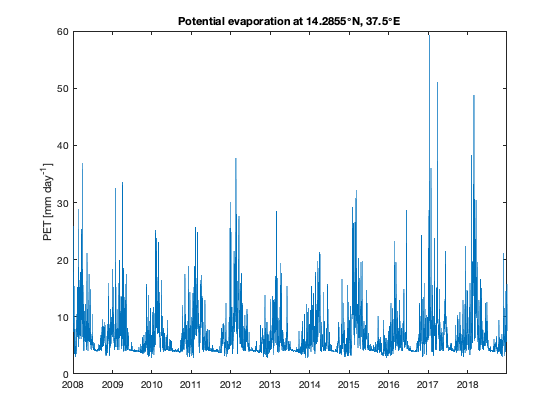# pet documentation

The pet gives the potential reference evapotranspiration following Hargreaves-Samani.

## Syntax

`pevap = pet(Ra,tmax,tmin,tmean)`

## Description

pevap = pet(Ra,tmax,tmin,tmean) computes the Hargreaves equation for the potential evapotranspiration (PET) for extraterrestrial radiation Ra (such as from the solar_radiation function), and temperature maxima, minima, and means. Temperatures tmax, tmin, and tmean can be vectors, or can be cubes in which the first two dimensions are spatial and the third dimension corresponds to time. The inputs tmax, tmin, and tmean represent maximinum, minimum, and mean temperatures over a given time period.

## Example

This example is an abbreviated form of the example given in the documentation for the spei function. You may wish to check out that page for a little more context and in-depth explanation.

Begin by loading data and converting units. Precipitation comes in units kg^2/m^2/s and we change these units to mm/day. Temperatures are in K and we change them to degrees C.

```load ncep-ncar

P    = P*3600*24;
T    = T-273.15;
TMAX = TMAX-273.15;
TMIN = TMIN-273.15;
```

## Calculate extraterrestrial solar radiation

Hargreaves and Samani's PET equation requires extraterrestrial radiation (Ra) as input. Ra is the solar radiation on a horizontal surface at the top of the Earth's atmosphere and is computed based on the orbital parameters of the Earth which are dependent on latitude. We wish to have the temporal variations of solar radiation integrated over daily time intervals which can be calculated using the function <solar_radiation_documentation.html solar_radiation function. Use meshgrid to turn the lat,lon vectors into grids, which will thereby give us a distinct latitude value for each grid cell:

```[Lon,Lat] = meshgrid(lon,lat);

% Plot grid cell 5,5:
plot(t,squeeze(Ra(5,5,:)))
ylabel('Ra [MJ m^2 day^{-1}]')
title(['Solar radiation at ' num2str(lat(5)) '\circN'])
```## Calculate potential evapotranspiration

SPEI requires precipitation and potential evapotranspiration (PET). PET is calculated based on the formula of Hargreaves and Samani (1985) which estimates reference crop evapotranspiration based on temperature and extraterrestrial radiation.

There are numerous approaches to calculating potential evaporation. A compilation is found here (see supplements). Here we use the formula of Hargreaves-Samani (1985) which is a temperature based method to calculate the potential evapotranspiration (PET) and is implemented in the function pet. The main advantage of using the Hargreaves-Samani equation lies in its simplicity and low requirements regarding input parameters.

```pevap = pet(Ra,TMAX,TMIN,T);

plot(t,squeeze(pevap(5,5,:)))
ylabel('PET [mm day^{-1}]')
title(['Potential evaporation at ' num2str(lat(5)) '\circN, ' num2str(lon(5)) '\circE' ])
%
```## References

• Hargreaves, George H., and Zohrab A. Samani. "Reference crop evapotranspiration from temperature." Applied Engineering in Agriculture 1.2 (1985): 96-99. doi:10.13031/2013.26773.
• Samani, Zohrab. "Estimating solar radiation and evapotranspiration using minimum climatological data." Journal of Irrigation and Drainage Engineering 126.4 (2000): 265-267. 10.1061/(ASCE)0733-9437(2000)126:4(265).

## Author Info

This function and supporting documentation were written by José Delgado and Wolfgang Schwanghart (University of Potsdam), February 2019, for the Climate Data Toolbox for Matlab.